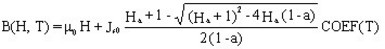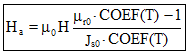# Analytic saturation curve + knee adjustment * exponential function of T

## Presentation

This model defines a B(H) behavior law:

• nonlinear (taking the saturation and the knee adjustment into consideration)
• for an isotropic material
• by means of a value of the saturation magnetic polarization Js and a value of the relative permeability μr, which decrease in an exponential way when the temperature increases.

## Mathematical model

This model consists, like the previous one, of a combination of a straight line and a curve. A coefficient adjusts the shape of the curve knee for a better approximation of the experimental curve. With this model, the saturation magnetization Js and the relative permeability μr decrease in an exponential way when the temperature increases (Js0 and μr are multiplied by a temperature coefficient COEF(T)).

The corresponding mathematical formula is written:with:where:

• μ0 is the permeability of vacuum; μ0 = 4 π 10-7 [H/m]
• μr0 is the relative permeability (at the origin) for T=0°C
• Js0 is the saturation magnetic polarization for T = 0°C [Tesla]
• a is the knee adjustment coefficient ( 0 < a and a < > 1 )

The smaller the coefficient, the sharper the knee is.

• COEF(T) is the previously described temperature coefficient

The shape of B(H) curve is presented in the figure below.## Example

An example of B(H) curve dependent on T is presented in the figure below.

It is an analytic curve of saturation + knee adjustment * exponential function of T with: Tcurie = 720°, C = 500°C